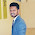# In this section of the blog we will learn how to use pandas for data analysis. You can think of pandas as an extremely powerful version of Excels, with a lot more features. In this section of the course, you should go through the notebooks in this order for this please ope below drive link:

## what is pandas in python ?

In computer programming language, pandas is a library written for the Python language for data manipulation and analysis the data.It's also performed the data structures and operation for manipulating numerical table and times series data.
Some Latest important update about pandas :
What’s new in 1.0.5 (June 17, 2020)

### How to install pandas in python ?

WE USE THIS FOLLOWING COMMAND LINE FOR INSTALL PANDAS
pip install pandas !

#### How to import pandas  in python ?

WE USE THIS FOLLOWING COMMAND LINE FOR import  PANDAS
import pandas as pd !

#### Introduction to Pandas Series DataFrames Missing Data GroupBy Merging,Joining,and Concatenating Operations Data Input and Output SOME EXAMPLE ON PANDAS: 3.1 example: Let us now create an indexed DataFrame using array. ``` import pandas as pd data = {'Name':['sachin', 'pagar', 'sunil', 'Rahul'],'Age':[28,34,29,42]} df = pd.DataFrame(data, index=['rank1','rank2','rank3','rank4']) print df ``` Its output is as follows − ``` Age Name rank1 28 sachin rank2 34 pagar rank4 42 Rahul rank3 29 Sunil ``` 3.2 example: ``` import pandas as pd data = {'Name':['sachin', 'ram', 'sham', 'gokul'],'Age':[28,34,29,42]} df = pd.DataFrame(data) print df ``` Its output is as follows − ``` Age Name 0 28 sachin 1 34 ram 3 42 gokul 2 29 Sham ``` 3.3 Example: ``` import pandas as pd data = [['Alisha',10],['Ashwini',12],['Apeksha',13]] df = pd.DataFrame(data,columns=['Name','Age'],dtype=float) print df ``` Its output is as follows − ``` Name Age 0 Alisha 10.0 1 Ashwini 12.0 2 Apeksha 13.0 ``` Some FAQ on Pandas 1. Why Pandas are used in Python? In simple way Pandas is a Library in Computer Programming. It is written for the support programming in  Python Programming Language . With the help of python library we do the programming work for data analysis and manipulation.In the simpler word, Pandas help us  putting the data in a tabular form. 2. What is NumPy and pandas in Python? What is Numpy? Similar to pandas, Numpy is one of the most widely used python library in data science. It provide high-performance to us, easy to use structure and data analysis tool in python. Unlike Pandas library which provides object for the multi-dimensional array, Pandas provides in-memory table objects called Dataframe object. 3. What is difference between Numpy and pandas? Key Differences: Pandas library which provides object for the multi-dimensional array, Pandas provides in-memory table objects called Dataframe object whereas library which provides object for the multi-dimensional array, Pandas provides in-memory table objects called Dataframe object. 4. Is Numpy faster than pandas? From the different observation on function function, the NumPy array can be significantly performed the faster  operations than Pandas series. NumPy array can be used in place of Pandas serie if the more functionality offered by Pandas series is not critical. See the Package overview for more detail about what’s in the librarys. What’s new in 0.25.3 (October 31, 2019) Installation Useful link: Binary Installersfig 01) pandas in python tutorials-beginners Tags: pandas in python tutorials-beginners,Is Numpy faster than pandas,What is difference between Numpy and pandas,What is NumPy and pandas in Python,Why Pandas are used in Python CLICK HERE OR  https://drive.google.com/open?id=1RKv0v0V9kfvMceWb2QHdWfavDffsGAgV

Previous
Next Post »

Andy333